5G时代下一个聚合的编程学习网

# 十大排序算法详解

## 引言

• 基于比较的排序算法可以细分为：
• 基于交换类：冒泡排序、快速排序
• 基于插入类：直接插入排序、希尔排序
• 基于选择类：简单选择排序、堆排序
• 基于归并类：归并排序
• 基于非比较的排序算法可以分为：桶排序、计数排序和基数排序。也有人将排序归纳为8大排序，原因是基数排序和计数排序是建立在桶排序之上或者是一种特殊的桶排序，但是计数排序和基数排序有它们各自的特征。

## 比较类排序

### 交换类

#### 冒泡排序

##### 主要思想

• 从第一个元素开始往后遍历，每到一个位置判断是否比后面的元素大，如果比后面元素大，那么就交换两者大小，然后继续向后，这样的话进行一轮之后就可以保证最大的那个数被交换交换到最末的位置可以确定
• 第二次同样从开始起向后判断着前进，如果当前位置比后面一个位置更大的那么就和他后面的那个数交换。但是有点注意的是，这次并不需要判断到最后，只需要判断到倒数第二个位置就行(因为第一次我们已经确定最大的在倒数第一，这次的目的是确定倒数第二)
• 同理，后面的遍历长度每次减一，直到第一个元素使得整个元素有序。
##### 代码
``````package com.base.sort.compare.exchange;

/**
* @Author yamon
* @Date 2021-08-01 15:22
* @Description
* @Version 1.0
*/
public class BubblingSort {
public void bubblingSort(int[] a){
for(int i = a.length-1;i>=0;i--){
for(int j = 0;j<i;j++){
if(a[j]>a[j+1]){
int temp = a[j];
a[j] = a[j+1];
a[j+1] =temp;
}
}
}
}

public static void main(String[] args) {
int[] arr ={2,5,1,4,3};
new BubblingSort().bubblingSort(arr);
}
}

``````

#### 快速排序

##### 基本思想：
• 快排需要将序列变成两个部分，就是序列左边全部小于一个数序列右面全部大于一个数，然后利用递归的思想再将左序列当成一个完整的序列再进行排序，同样把序列的右侧也当成一个完整的序列进行排序。
• 其中这个数在这个序列中是可以随机取的，可以取最左边，可以取最右边，当然也可以取随机数。但是通常不优化情况我们取最左边的那个数。

##### 代码
``````package com.base.sort.compare.exchange;

import java.util.Arrays;

/**
* @Author yamon
* @Date 2021-08-01 15:30
* @Description
* @Version 1.0
*/
public class QuickSort {
/**
* 快速排序算法
*
* @param a     待排序数组
* @param left  左边界
* @param right 右边界
*/
public void quickSort(int[] a, int left, int right) {
//重新赋值两个变量，这两个变量根据数组变化而变化，但是left和right不能变，分治的时候需要
int low = left;
int high = right;
//分治递归停止条件
if (low > high) {
return;
}
//首先取哨兵也就是第一个位置上的数字作为待比较的数字
int k = a[low];
while (low < high) {
while (low < high && a[high] >= k) {
high--;
}
//否则的话就需要交换两个数字
a[low] = a[high];
while (low < high && a[low] <= k) {
low++;
}
//否则的话，需要交换
a[high] = a[low];
}
//分治前的最后一步也需要
a[low] = k;
//开始分治
quickSort(a, left, low - 1);
quickSort(a, low + 1, right);
}

public static void main(String[] args) {
int[] arr = {5, 1, 3, 9, 8, 2, 6, 4, 7};
new QuickSort().quickSort(arr, 0, arr.length - 1);
System.out.println(Arrays.toString(arr));
}
}

``````

### 插入类

#### 直接插入排序

##### 基本思想

• 选取当前位置(当前位置前面已经有序) 目标就是将当前位置数据插入到前面合适位置。
• 向前枚举或者二分查找，找到待插入的位置。
• 移动数组，赋值交换，达到插入效果。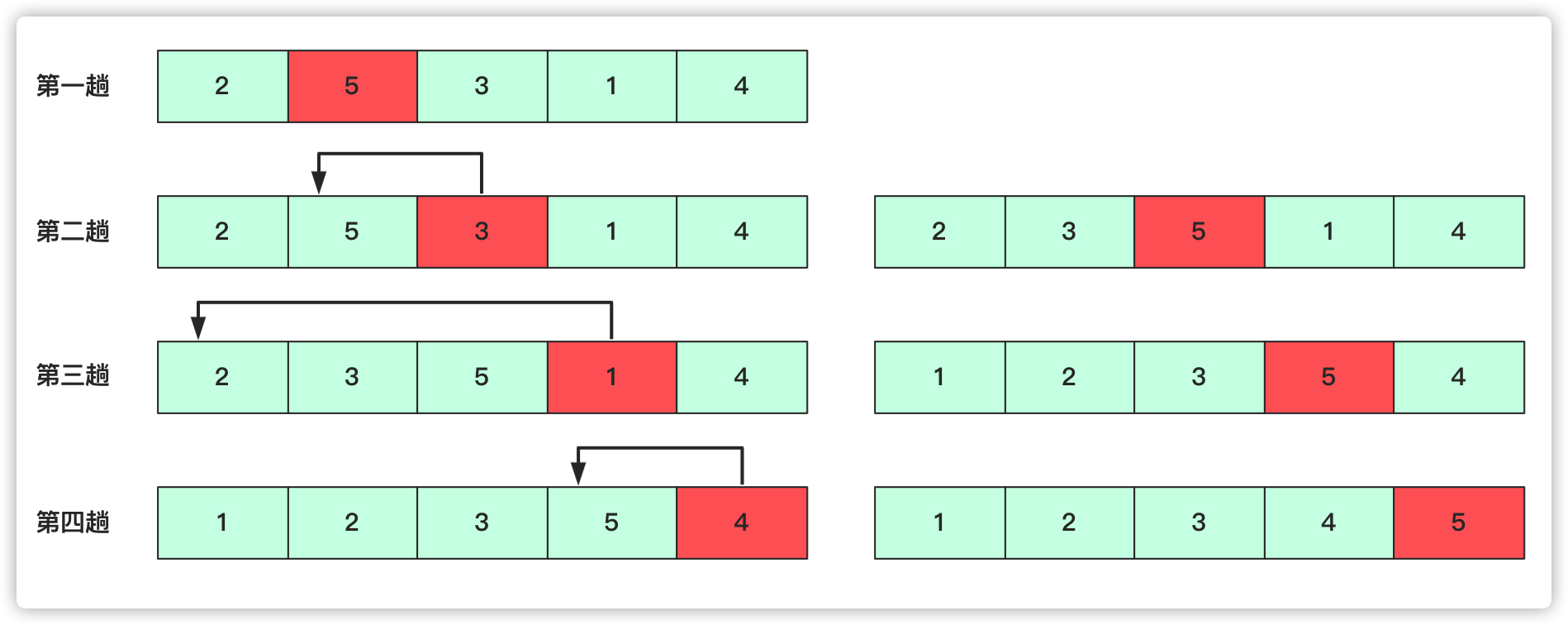##### 代码：
``````package com.base.sort.compare.exchange;

import java.util.Arrays;

/**
* @Author yamon
* @Date 2021-08-01 16:07
* @Description
* @Version 1.0
*/
public class InsertSort {
/**
* 简单插入排序
* @param a 待排序数组
*/
public void insertSort(int[] a){
int team;
for(int i = 1; i<a.length;i++){
System.out.println(Arrays.toString(a));
//首先将第1个位置上的数字赋值
team = a[i];
for(int j = i-1;j>=0;j--){
//这个数字和前一个数字进行比较，如果前一个数字大于这个数字，则交换。否则，进行下一个。
if(a[j]>team){
//
a[j+1] = a[j];
a[j] = team;
}else{
break;
}
}
}
}

public static void main(String[] args) {
int[] arr = {2,5,3,1,4};
new InsertSort().insertSort(arr);
System.out.println(Arrays.toString(arr));
}
}

``````

#### 希尔排序

##### 基本思想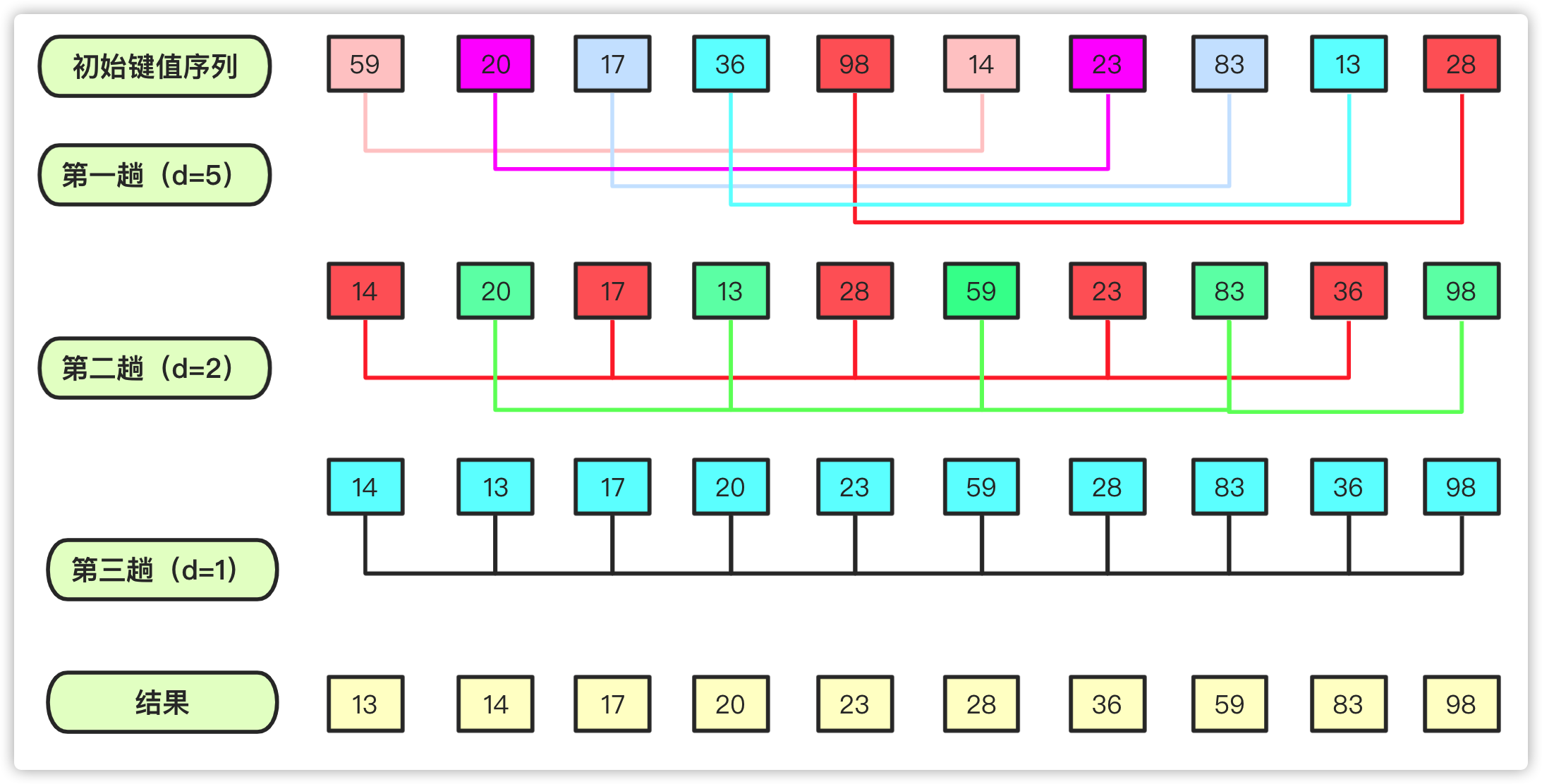##### 代码
``````package com.base.sort.compare.exchange;

import java.util.Arrays;

/**
* @Author yamon
* @Date 2021-08-01 16:22
* @Description
* @Version 1.0
*/
public class ShellSort {
public void shellSort(int[] a) {
int d = a.length;
//临时变量
int team = 0;
for (; d >= 1; d /= 2) {
//共分为d组,以下就是每个组内的插入排序了。
for (int i = d; i < a.length; i++) {
//到哪个元素就看这个元素在哪个组即可
team = a[i];
for (int j = i - d; j >= 0; j -= d) {
if (a[j] > team) {
a[j + d] = a[j];
a[j] = team;
} else {
break;
}
}
}
}
}

public static void main(String[] args) {
int[] arr = {59, 20, 17, 36, 98, 14, 23, 83, 13, 28};
new ShellSort().shellSort(arr);
System.out.println(Arrays.toString(arr));
}
}

``````

### 选择类排序

#### 简单选择排序

##### 简介以及基本思想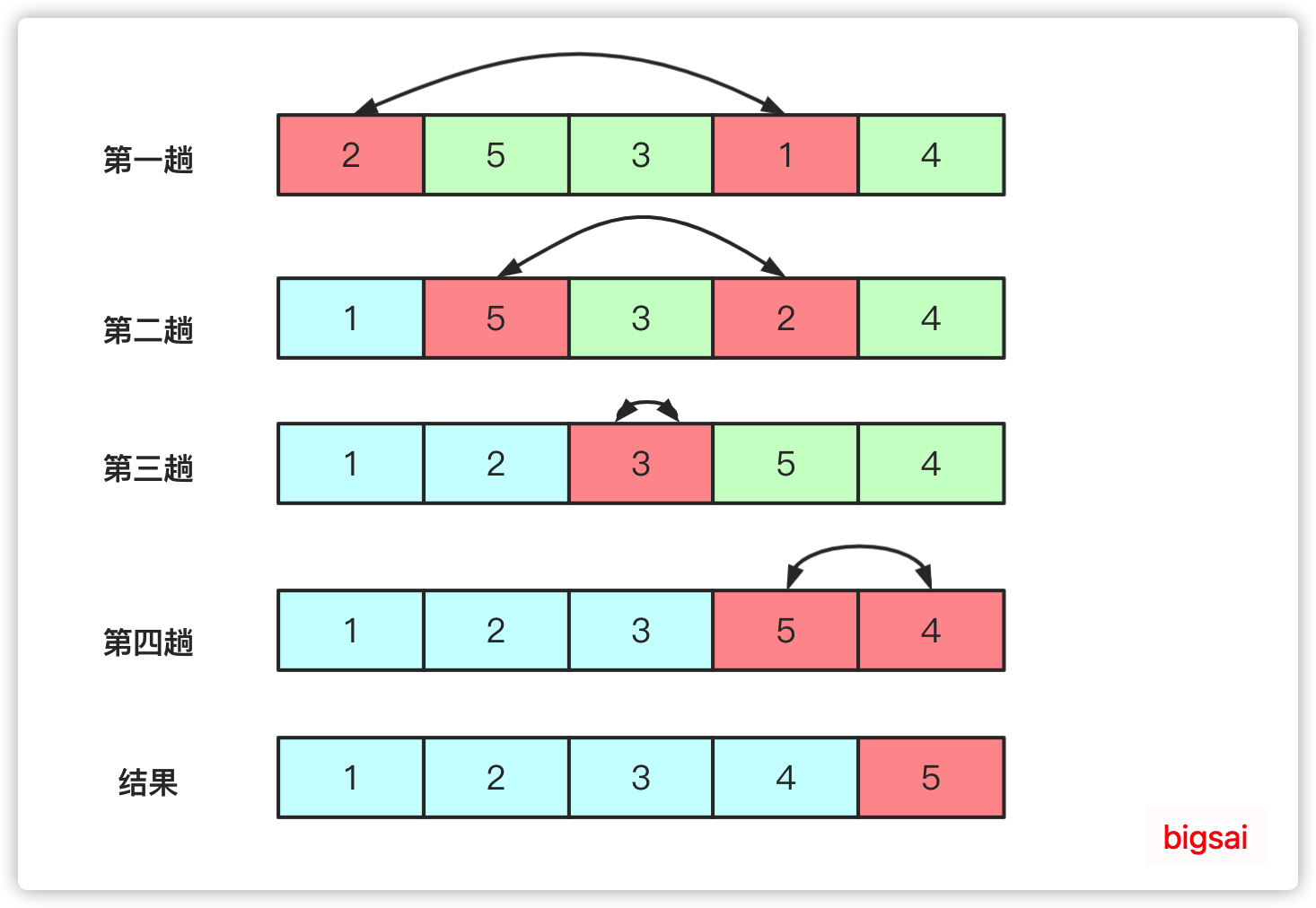##### 代码
``````package com.base.sort.compare.exchange;

import java.util.Arrays;

/**
* @Author yamon
* @Date 2021-08-01 16:36
* @Description
* @Version 1.0
*/
public class SelectSort {
public void selectSort(int[] a){
for(int i = 0;i<a.length-1;i++){
//定义最小位置
int min = i;
for(int j = i+1;j<a.length;j++){
//遍历后面的数字，跟第一个数字相比，找出最小的；
if(a[j]<a[min]){
min = j;
}
}
//一遍下来已经找到最小的，然后直接交换
if(min!=i){
//与第i个位置交换
swap(a, i, min);
}

}
}
public void swap(int[] a, int i, int j){
int temp = a[i];
a[i] = a[j];
a[j] = temp;
}

public static void main(String[] args) {
int[] arr ={2,5,3,1,4};
new SelectSort().selectSort(arr);
System.out.println(Arrays.toString(arr));
}
}

``````

#### 堆排序

• 如果所有节点大于孩子节点值，那么这个堆叫做大根堆，堆的最大值在根节点。
• 如果所有节点小于孩子节点值，那么这个堆叫做小根堆，堆的最小值在根节点。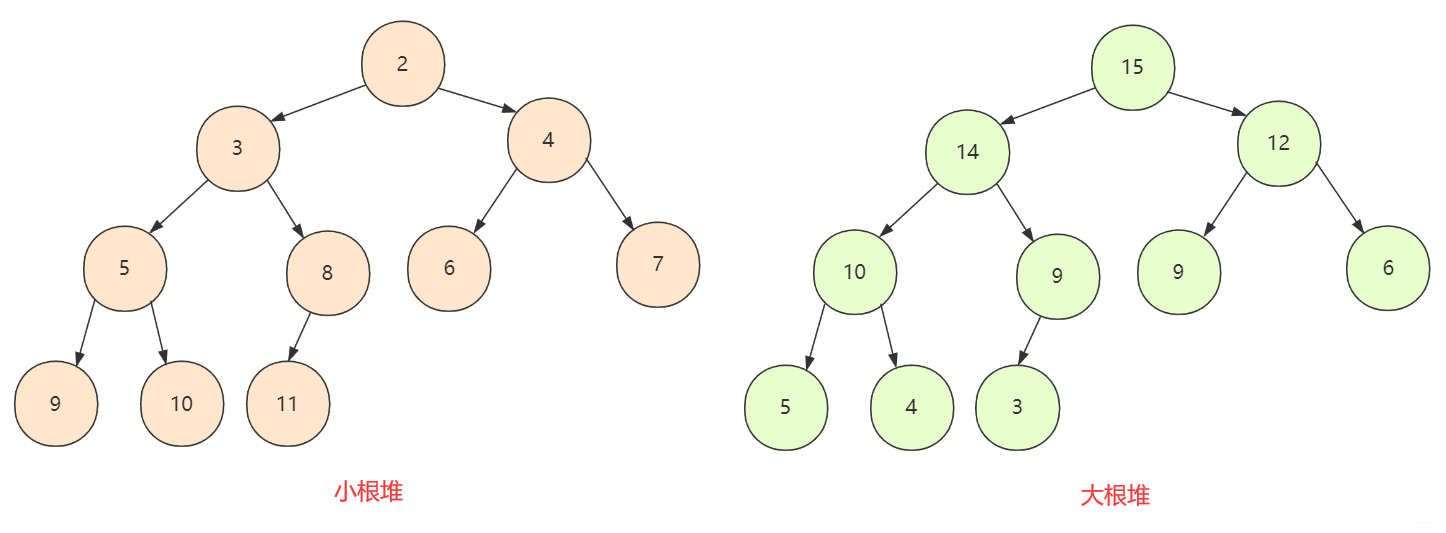##### 代码
``````package com.base.sort.compare.exchange;

import java.lang.reflect.Array;
import java.util.Arrays;

/**
* @Author yamon
* @Date 2021-08-01 17:01
* @Description
* @Version 1.0
*/
public class HeapSort {
/**
* 交换数组arr中m和n的位置
*
* @param arr 待交换数组
* @param m   数组下标为m
* @param n   数组下标为n
*/
public void swap(int[] arr, int m, int n) {
int temp = arr[m];
arr[m] = arr[n];
arr[n] = temp;
}

/**
* 用给定的数组创建成堆
*
* @param arr 给定的数组
*/
public void createHeap(int[] arr) {
for (int i = arr.length / 2; i >= 0; i--) {
shiftDown(arr, i, arr.length);
}
}

/**
* 下移交换，把当前节点有效变换成为一个堆（小根堆）
*
* @param arr   创建的数组
* @param index 线标
* @param len   长度
*/
public void shiftDown(int[] arr, int index, int len) {
//0号位置不需要
int leftChild = index * 2 + 1;
int rightChild = index * 2 + 2;
if (leftChild >= len) {
return;
} else if (rightChild < leftChild && arr[rightChild] < arr[index] && arr[rightChild] < arr[leftChild]) {
//交换节点值
swap(arr, index, rightChild);
//可能会对孩子节点的堆有影响，向下重构
shiftDown(arr, rightChild, len);
} else if (arr[leftChild] < arr[index]) {
//交换做孩子
swap(arr, index, leftChild);
shiftDown(arr, leftChild, len);
}
}

public void heapSort(int[] arr){
System.out.println("原数组为："+ Arrays.toString(arr));
//临时存储结果
int[] val = new int[arr.length];
//建堆
createHeap(arr);
System.out.println("建立堆之后的序列为："+ Arrays.toString(arr));
// 进行n次取值建堆，每次取堆顶元素放入val数组中，最终结果即为一个递增排序的序列
for(int i = 0;i<arr.length;i++){
//将堆顶放入结果中
val[i] = arr;
//删除堆顶元素，将末尾元素放到堆顶
arr = arr[arr.length-i-1];
//将这个堆调整为合法的小根堆，主义长度上的变化
shiftDown(arr, 0, arr.length-i);
}
//数值克隆
for(int i = 0;i<arr.length;i++){
arr[i] = val[i];
}
System.out.println("堆排序后的序列为:"+Arrays.toString(arr));
}

}

``````

### 归并类排序

#### 归并排序

##### 基本思想

• 第一次：整串先进行划分成一个一个单独，第一次是将序列中(`1 2 3 4 5 6---`)两两归并成有序，归并完(`xx xx xx xx----`)这样局部有序的序列。
• 第二次就是两两归并成若干四个(`1 2 3 4 5 6 7 8 ----`)每个小局部是有序的
• 就这样一直到最后这个串串只剩一个，然而这个耗费的总次数logn。每次操作的时间复杂的又是`O(n)`。所以总共的时间复杂度为`O(nlogn)`.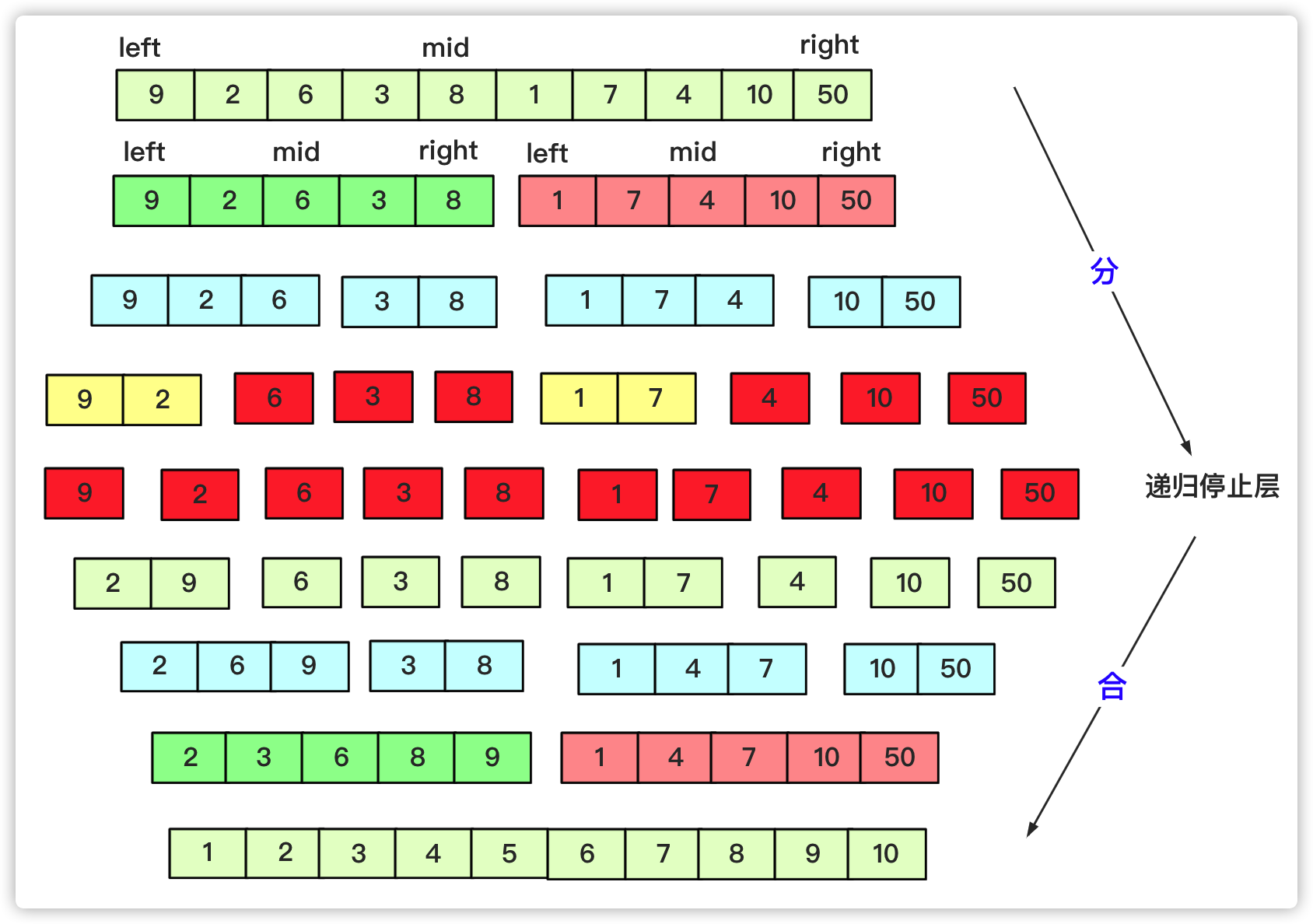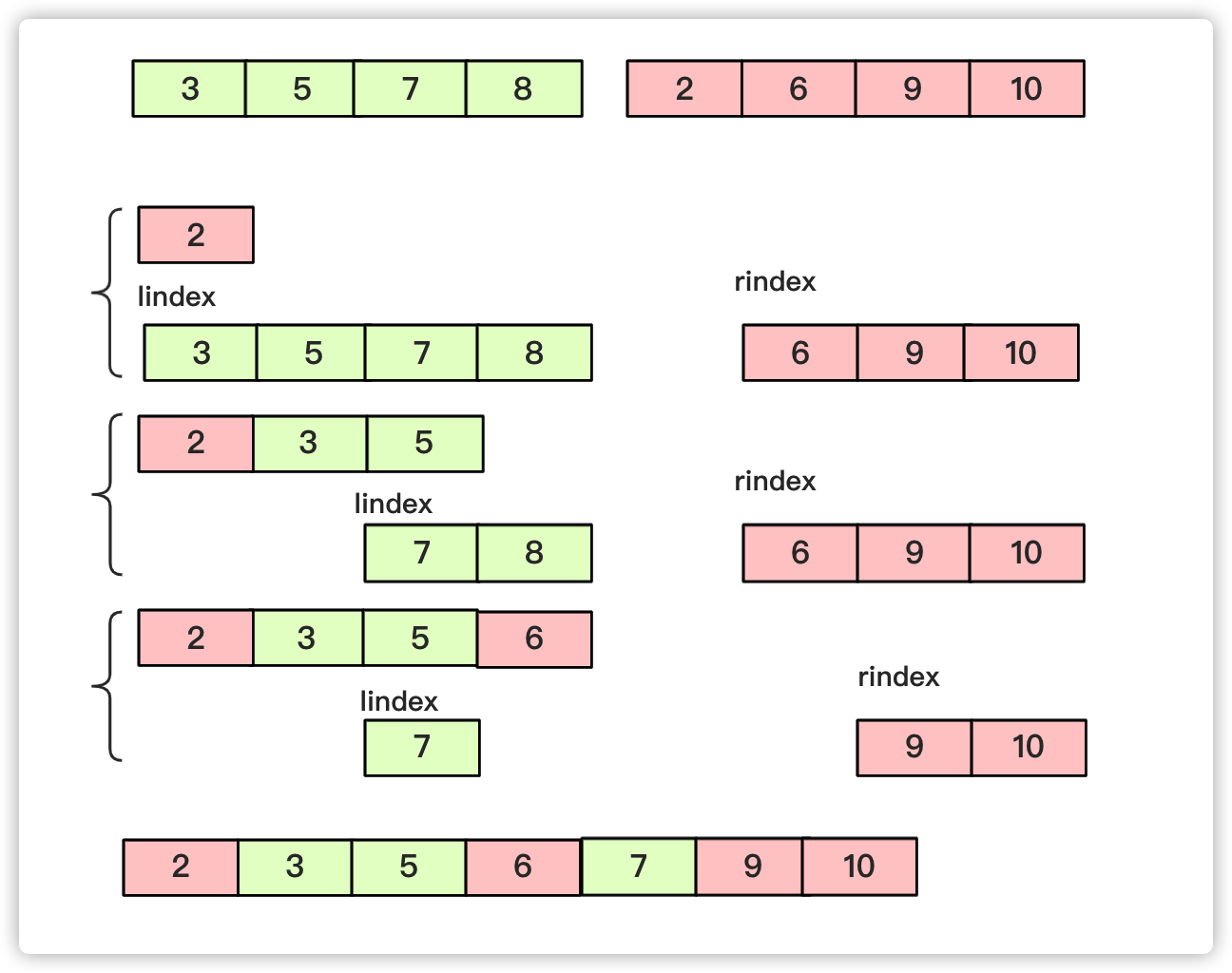##### 代码
``````package com.base.sort.compare.exchange;

import java.util.Arrays;

/**
* @Author yamon
* @Date 2021-08-02 15:59
* @Description 归并排序
* @Version 1.0
*/
public class MergeSort {
public void mergeSort(int[] arr, int left, int right) {
int mid = (left + right) / 2;
if (left<right){
//复杂度o(logn)
mergeSort(arr, left, mid);
mergeSort(arr, mid+1, right);
//o(n)
merge(arr, left, mid, right);
}

}
public void merge(int[] arr, int l, int mid, int r){
int lindex = l, rindex = mid+1;
int team[] = new int[r-l+1];
int teamIndex = 0;
while (lindex <= mid && rindex<=r){
//先左右比较
if(arr[lindex]<=arr[rindex]){
team[teamIndex++] = arr[lindex++];
}else {
team[teamIndex++] = arr[rindex++];
}
}
while (lindex<=mid){
//当一个越界后剩余按序号添加即可
team[teamIndex++] = arr[lindex++];
}
while (rindex<=r){
team[teamIndex++] = arr[rindex++];
}

for(int i = 0;i<teamIndex;i++){
arr[l+i] = team[i];
}
}

public static void main(String[] args) {
int[] arr = {9,2,6,3,8,1,7,4,10,60};
new MergeSort().mergeSort(arr, 0, arr.length-1);
System.out.println(Arrays.toString(arr));
}
}

``````

## 非比较类排序

### 桶类排序

#### 桶排序

##### 简介

• 桶：若干个桶，说明此类排序将数据放入若干个桶中。
• 桶：每个桶有容量，桶是有一定容积的容器，所以每个桶中可能有多个元素。
• 桶：从整体来看，整个排序更希望桶能够更匀称，即既不溢出(太多)又不太少。
##### 基本思想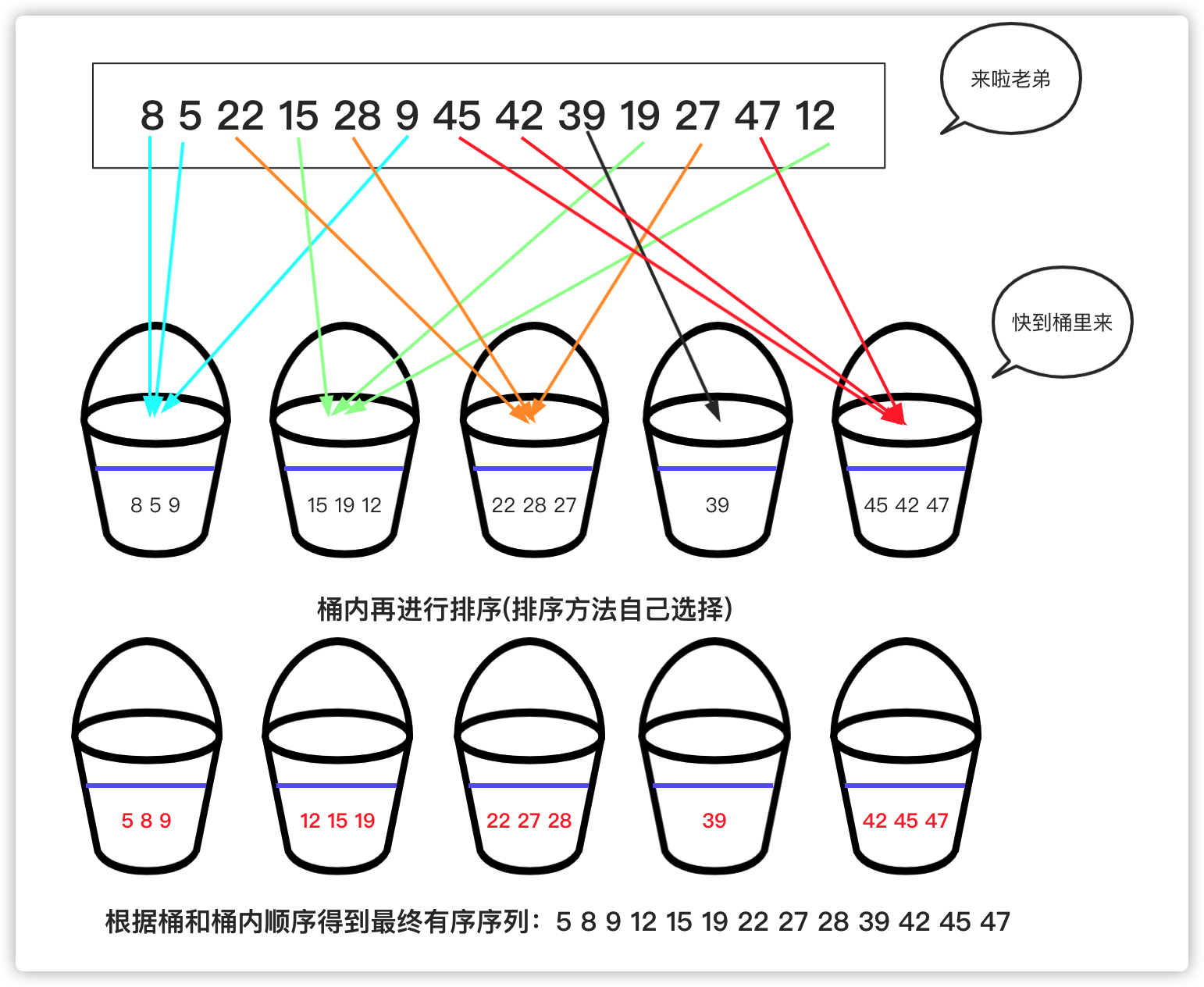##### 代码

``````package com.base.sort.compare.nonExchange;

import javax.xml.bind.helpers.AbstractUnmarshallerImpl;
import java.util.ArrayList;
import java.util.List;

/**
* @Author yamon
* @Date 2021-08-02 16:38
* @Description
* @Version 1.0
*/
public class BucketSort {
public void bucketSort(int[] arr){
List<List<Integer>> buckets = new ArrayList<>();
for(int i = 0;i<arr.length;i++){
}
//首先，将数组中的元素加入到桶中
for (int item : arr) {
//桶号
int index = item / 10;
//将数字添加到对应的桶号中
}
//对每个桶内进行排序
for (List<Integer> bucket : buckets) {
bucket.sort(null);
for (int j = 0; j < bucket.size(); j++) {
//打印输出
System.out.println(bucket.get(j) + " ");
}
}
}

public static void main(String[] args) {
int[] arr = {8, 15,22,30, 1, 4,29, 80,90, 92,85,36};
new BucketSort().bucketSort(arr);
}
}

``````

#### 计数排序

##### 基本思想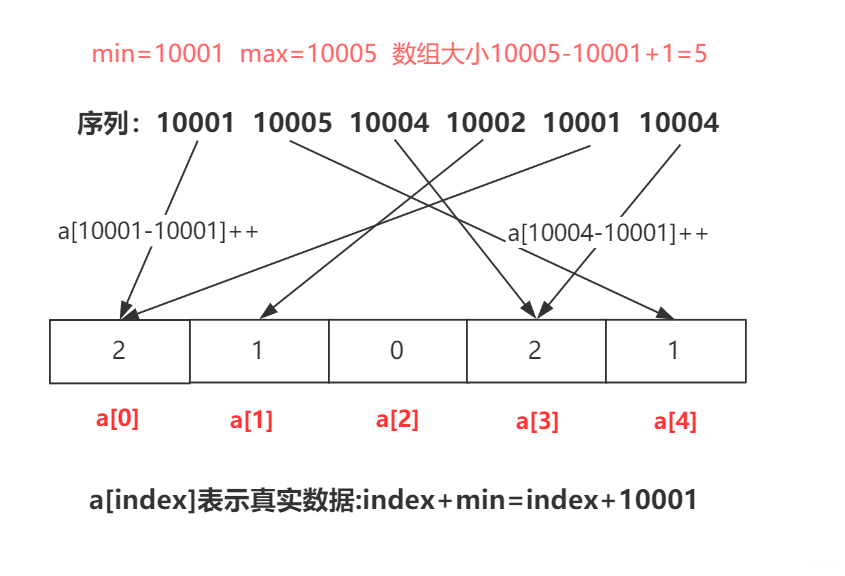##### 代码
``````package com.base.sort.compare.nonExchange;import java.util.Arrays;/** * @Author yamon * @Date 2021-08-02 16:58 * @Description * @Version 1.0 */public class CountSort {    public void countSort(int[] arr) {        int min = Integer.MAX_VALUE, max = Integer.MIN_VALUE;        for (int i = 0; i < arr.length; i++) {            if (arr[i] < min) {                min = arr[i];            }            if (arr[i] > max) {                max = arr[i];            }        }        //创建新的数组        int[] count = new int[max - min + 1];        for (int i = 0; i < arr.length; i++) {            count[arr[i] - min]++;        }        //排序取值        int index =0;        for(int i = 0;i<count.length;i++){            while (count[i]-->0){                arr[index++] = i+min;            }        }    }    public static void main(String[] args) {        int[] arr = {5,3,1,2,8,7,9,4};        new CountSort().countSort(arr);        System.out.println(Arrays.toString(arr));    }}
``````

#### 基数排序

##### 简介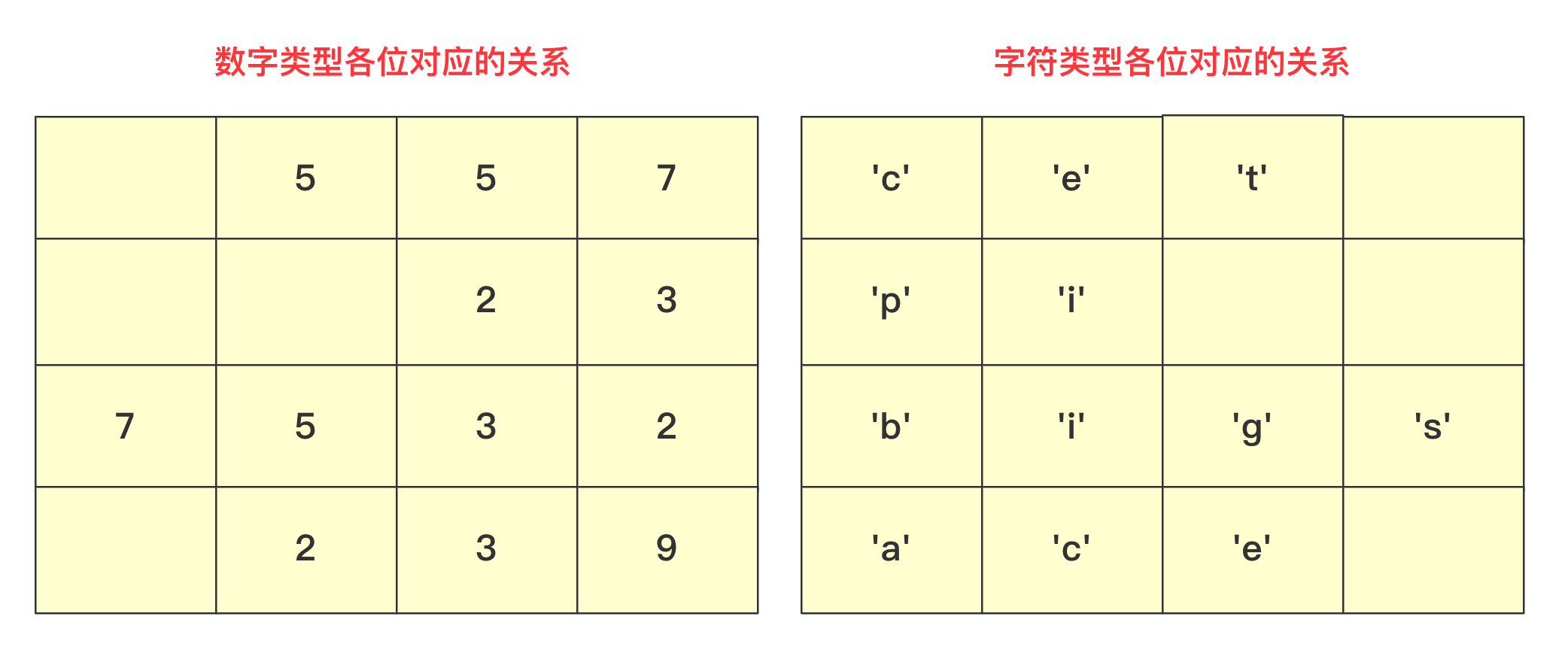##### 基本思想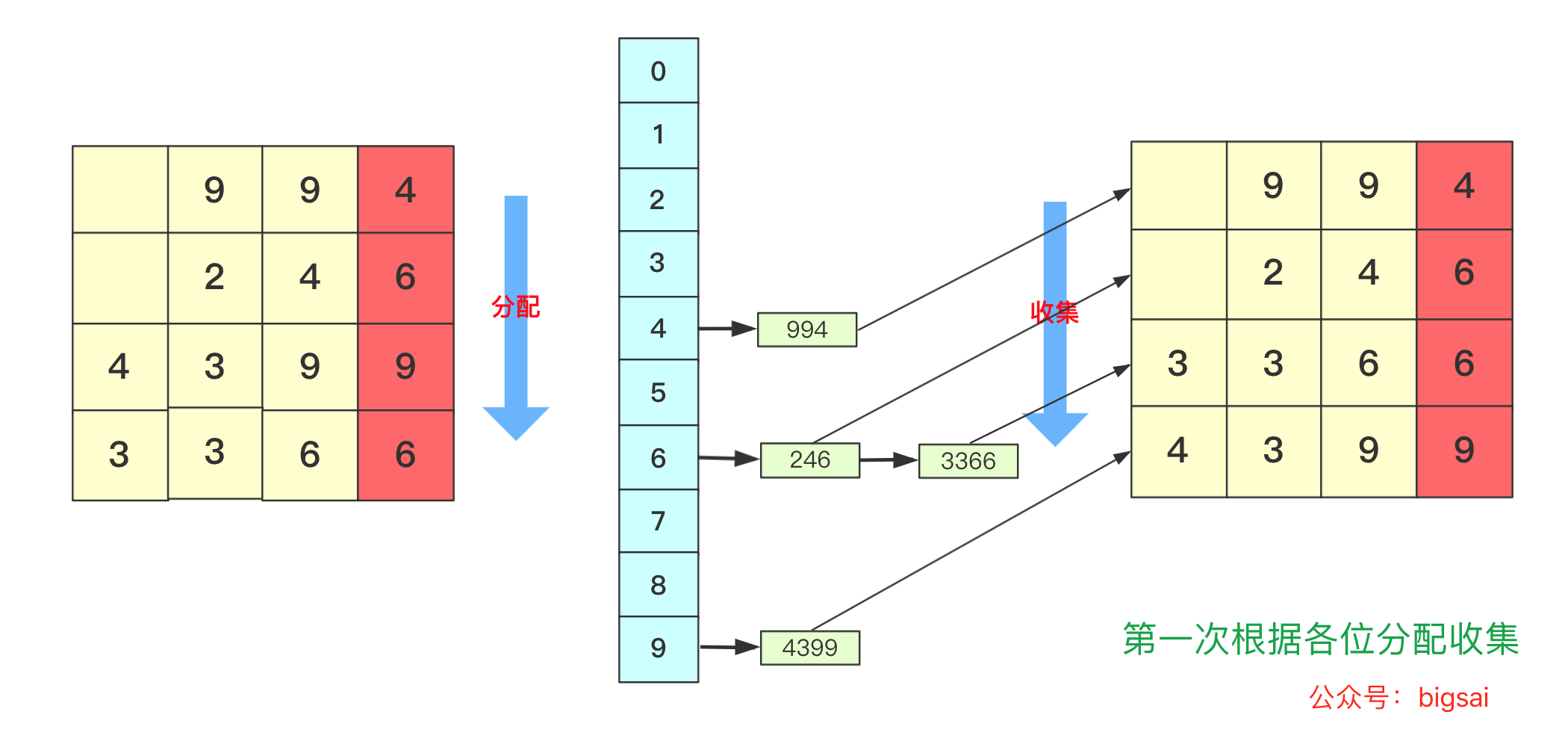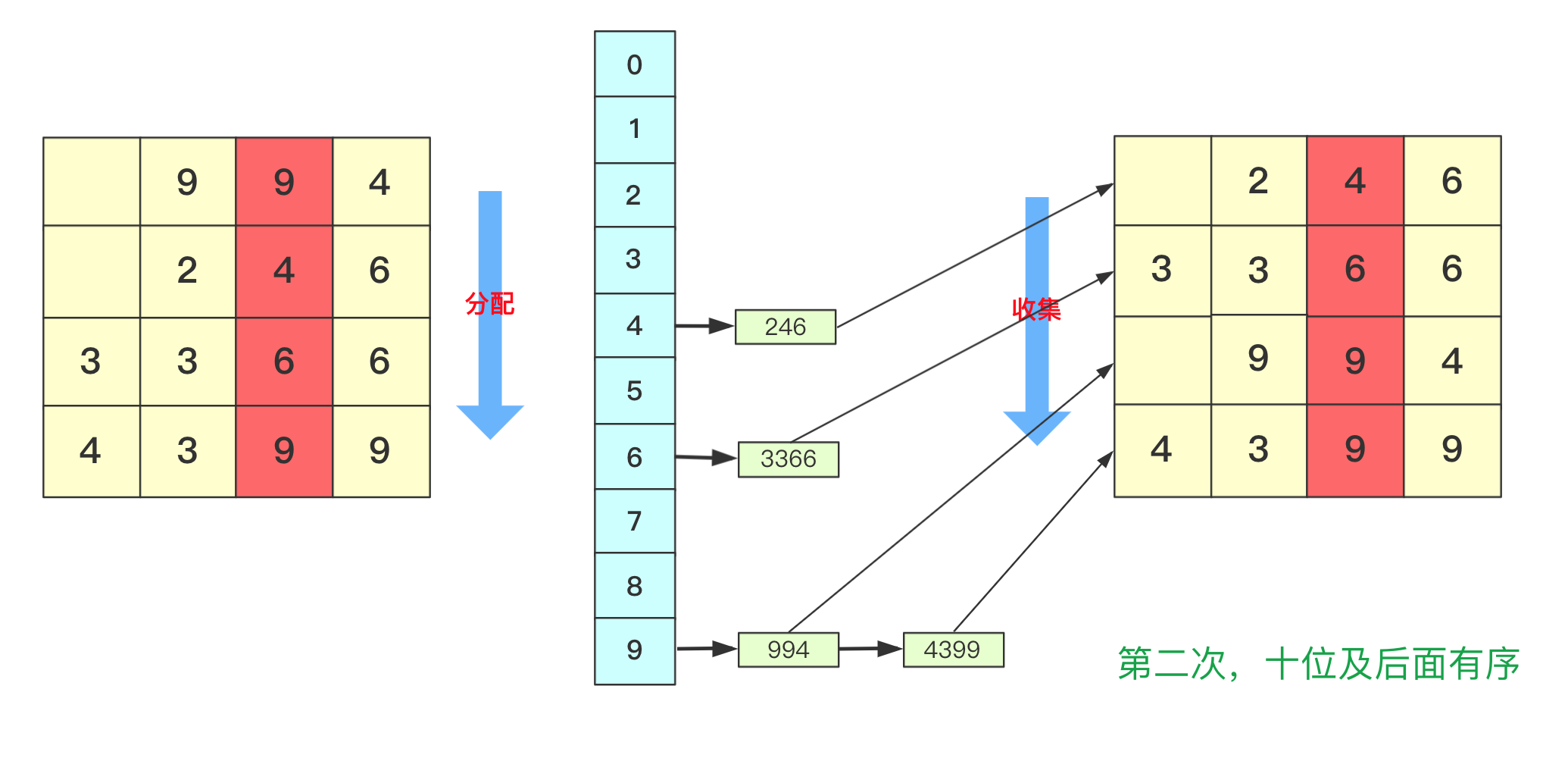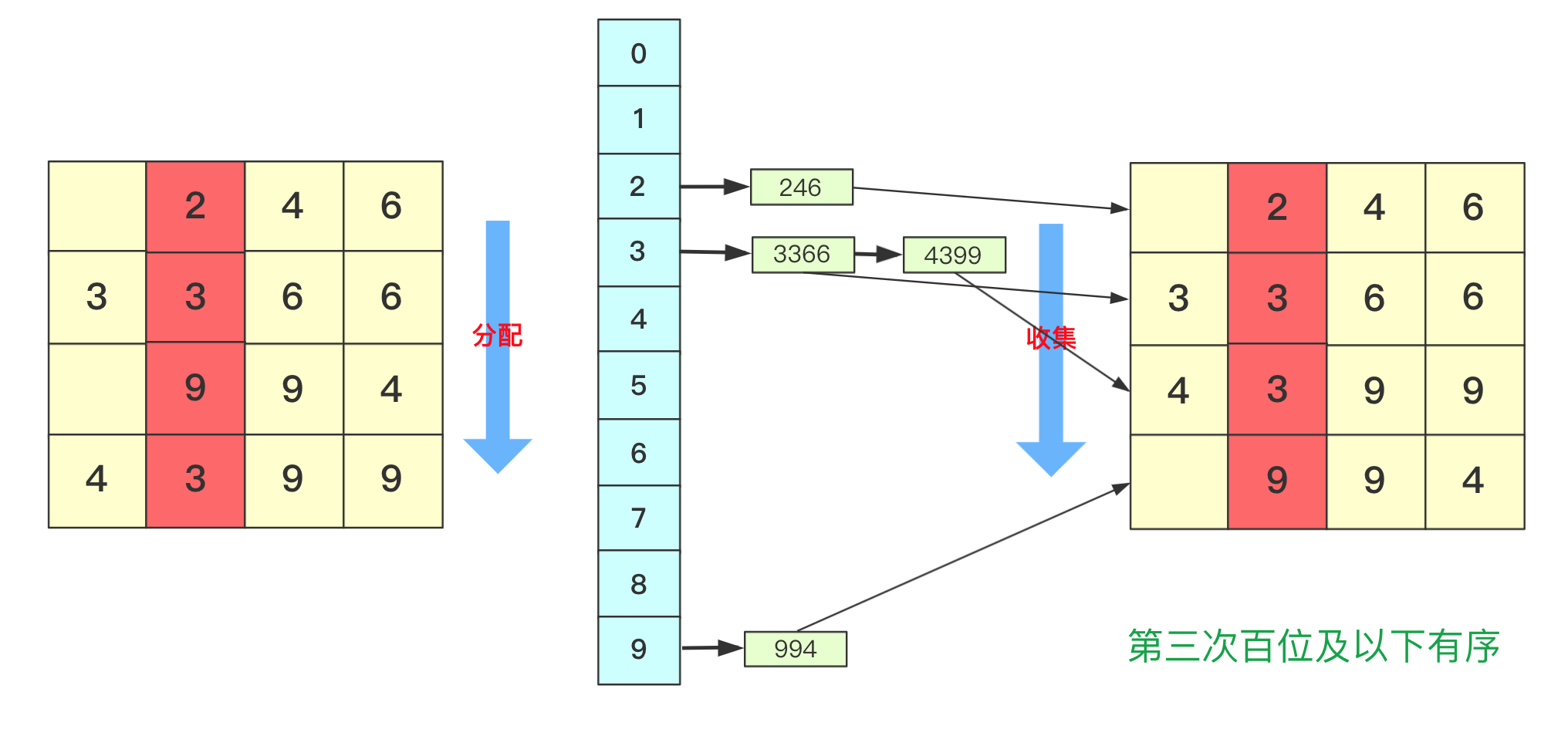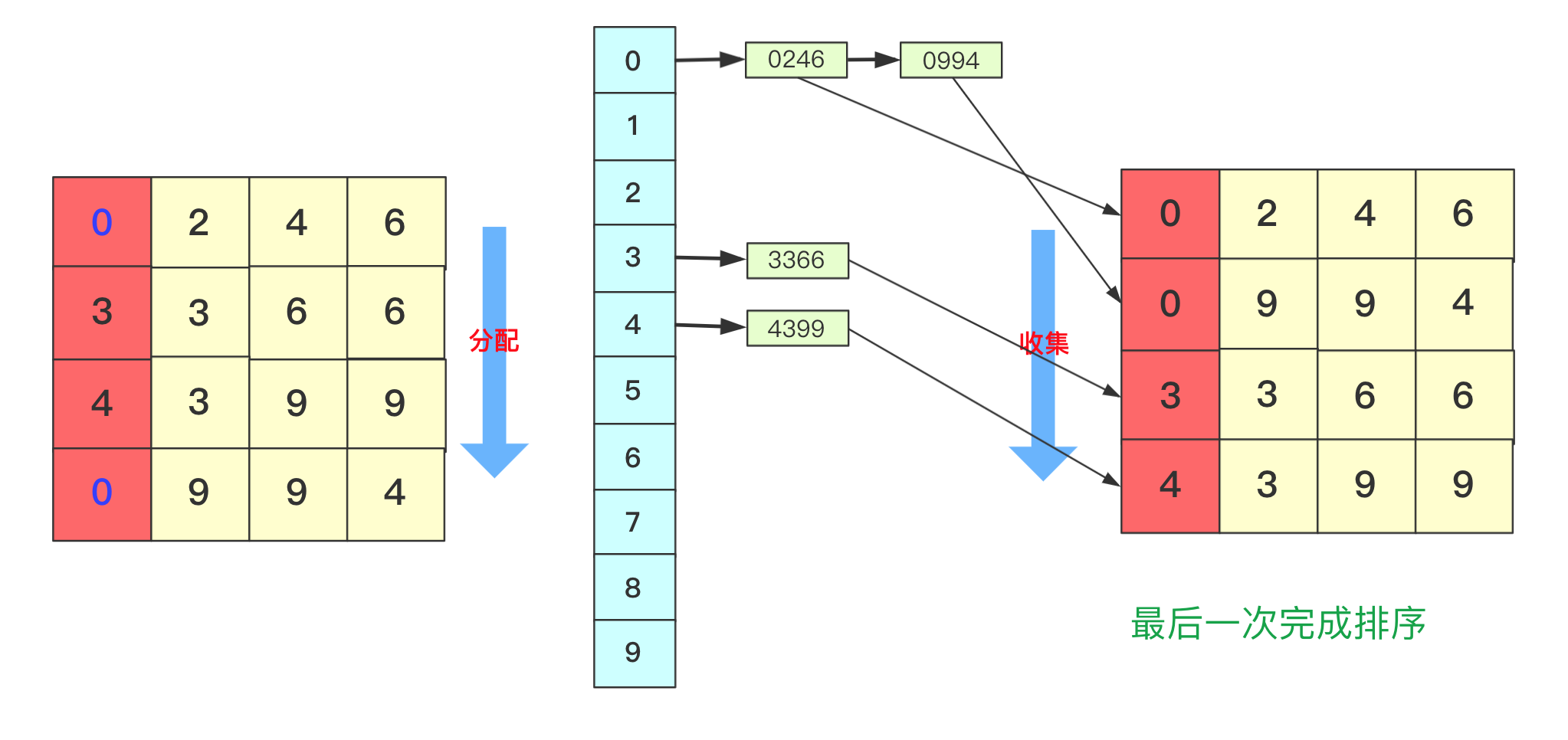##### 代码
``````package com.base.sort.compare.nonExchange;import java.util.ArrayList;import java.util.Arrays;import java.util.List;/** * @Author yamon * @Date 2021-08-02 17:37 * @Description 基数排序 * @Version 1.0 */public class RadixSort {    public void radixSort(int[] arr) {        List<Integer>[] bucket = new ArrayList;        //初始化bucket        for (int i = 0; i < 10; i++) {            bucket[i] = new ArrayList<>();        }        //找到最大值        int max = Integer.MIN_VALUE;        for (int i = 0; i < arr.length; i++) {            if (arr[i] > max) {                max = arr[i];            }        }        //1,10,100，1000.。。用来求对应位的数字        int divideNum = 1;        while (max > 0) {            //max 和num控制            for (int num : arr) {                //分配，将对应位置的数字分给对应的bucket                bucket[(num/divideNum)%10].add(num);            }            divideNum *= 10;            max/=10;            int idx = 0;            //收集 重新捡起数据            for(List<Integer> list: bucket){                for(int num:list){                    arr[idx++] = num;                }                //收集结束之后，需要清空下次接着使用。                list.clear();            }        }    }    public static void main(String[] args) {        int[] arr = {249, 994, 3399, 7898};        new RadixSort().radixSort(arr);        System.out.println(Arrays.toString(arr));    }}
``````

## 排序详细信息

GitHub网站 https://github.com/yamonc